Question

# 6. (4 pts) Consider the double integral∫R(x2+y)dA=∫10∫y−y(x2+y)dxdy+∫√21∫√2−y2−√2−y2(x2+y)dxdy.(a) Sketch the region of integration R in Figure 3.(b)...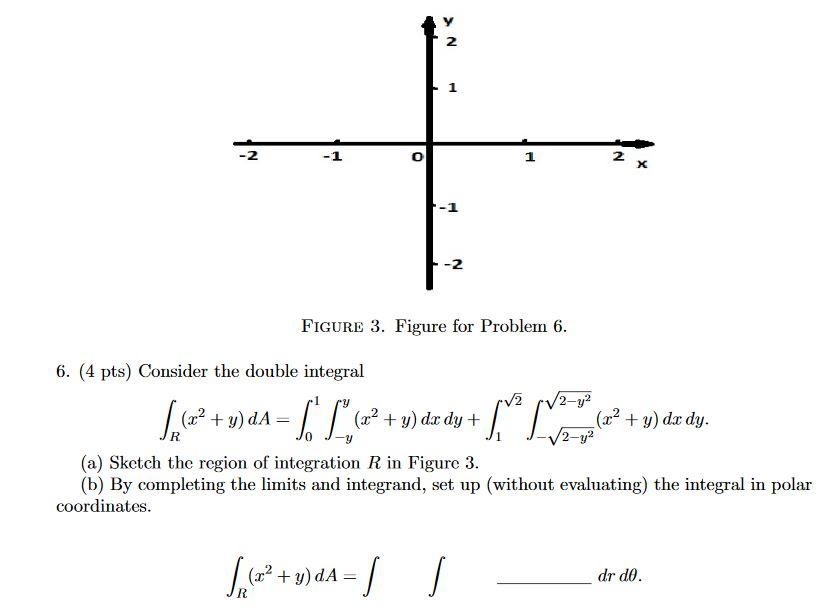6. (4 pts) Consider the double integral∫R(x2+y)dA=∫10∫y−y(x2+y)dxdy+∫√21∫√2−y2−√2−y2(x2+y)dxdy.(a) Sketch the region of integration R in Figure 3.(b) By completing the limits and integrand, set up (without evaluating) the integral in polar coordinates.

2 1 2 X -2 FIGURE 3. Figure for Problem 6. 6. (4 pts) Consider the double integral V2 2-y2 (2? + y) dA= (32 + y) dx dy + (x2 + y) dx dy. 2-y? (a) ketch the region of integration R in Figure 3. (b) By completing the limits and integrand, set up (without evaluating) the integral in polar coordinates. 5. (32 + y)dA= | dr do. -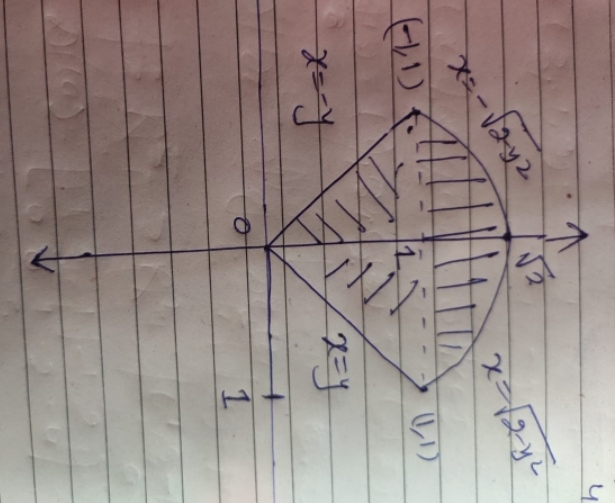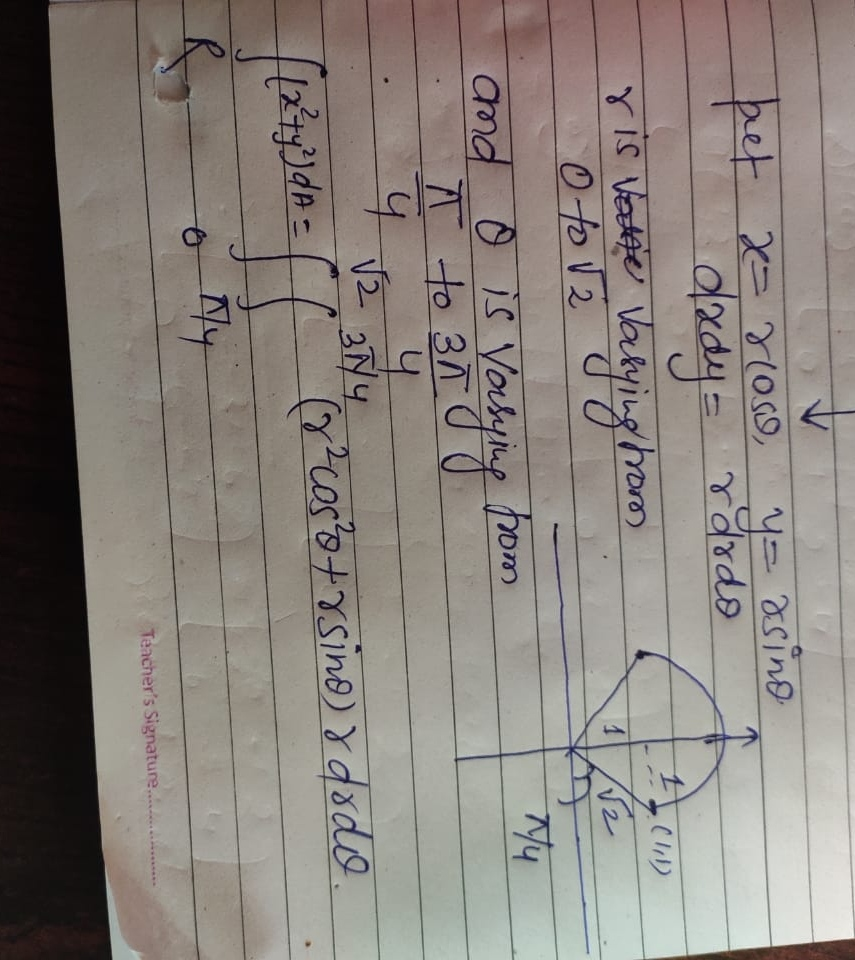#### Earn Coins

Coins can be redeemed for fabulous gifts.

Similar Homework Help Questions
• ### 6. (4 pts) Consider the double integral∫R(x2+y)dA=∫10∫y−y(x2+y)dxdy+∫√21∫√2−y2−√2−y2(x2+y)dxdy.(a) Sketch the region of integration R in Figure 3.(b)...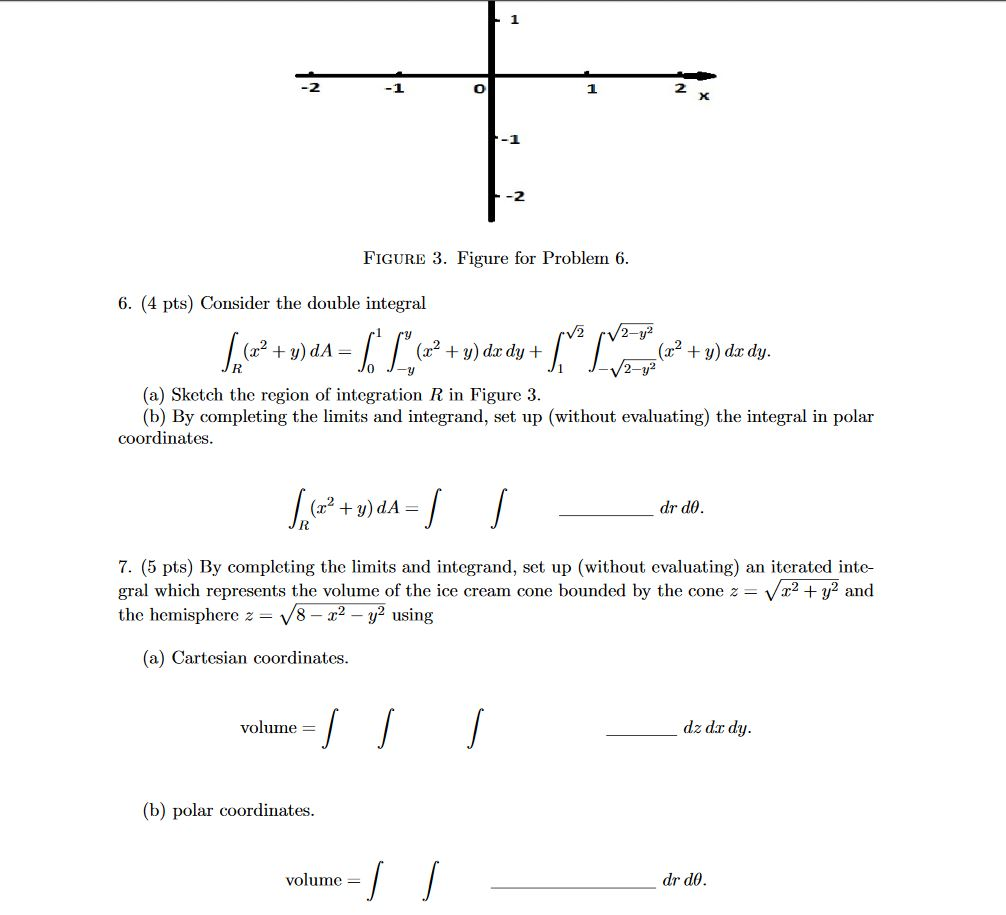6. (4 pts) Consider the double integral∫R(x2+y)dA=∫10∫y−y(x2+y)dxdy+∫√21∫√2−y2−√2−y2(x2+y)dxdy.(a) Sketch the region of integration R in Figure 3.(b) By completing the limits and integrand, set up (without evaluating) the integral in polar coordinates.∫R(x2+y)dA=∫∫drdθ.7. (5 pts) By completing the limits and integrand, set up (without evaluating) an iterated inte-gral which represents the volume of the ice cream cone bounded by the cone z=√x2+y2andthe hemisphere z=√8−x2−y2using(a) Cartesian coordinates.volume =∫∫∫dz dxdy.(b) polar coordinates.volume =∫∫drdθ. -1 -2 FIGURE 3. Figure for Problem 6. 6. (4 pts)...

• ### 6. (4 pts) Consider the double integral∫R(x2+y)dA=∫10∫y−y(x2+y)dxdy+∫√21∫√2−y2−√2−y2(x2+y)dxdy.(a) Sketch the region of integrationRin Figure 3.(b) By completing...6. (4 pts) Consider the double integral∫R(x2+y)dA=∫10∫y−y(x2+y)dxdy+∫√21∫√2−y2−√2−y2(x2+y)dxdy.(a) Sketch the region of integrationRin Figure 3.(b) By completing the limits and integrand, set up (without evaluating) the integral in polar coordinates. -1 -2 FIGURE 3. Figure for Problem 6. 6. (4 pts) Consider the double integral V2 /2-y² + = (x2 + y) dx dy + + y) do dy. 2-y2 (a) Sketch the region of integration R in Figure 3. (b) By completing the limits and integrand, set up (without evaluating)...

• ### 1 -1 O 1 2 x FIGURE 3. Figure for Problem 6. 6. (4 pts) Consider...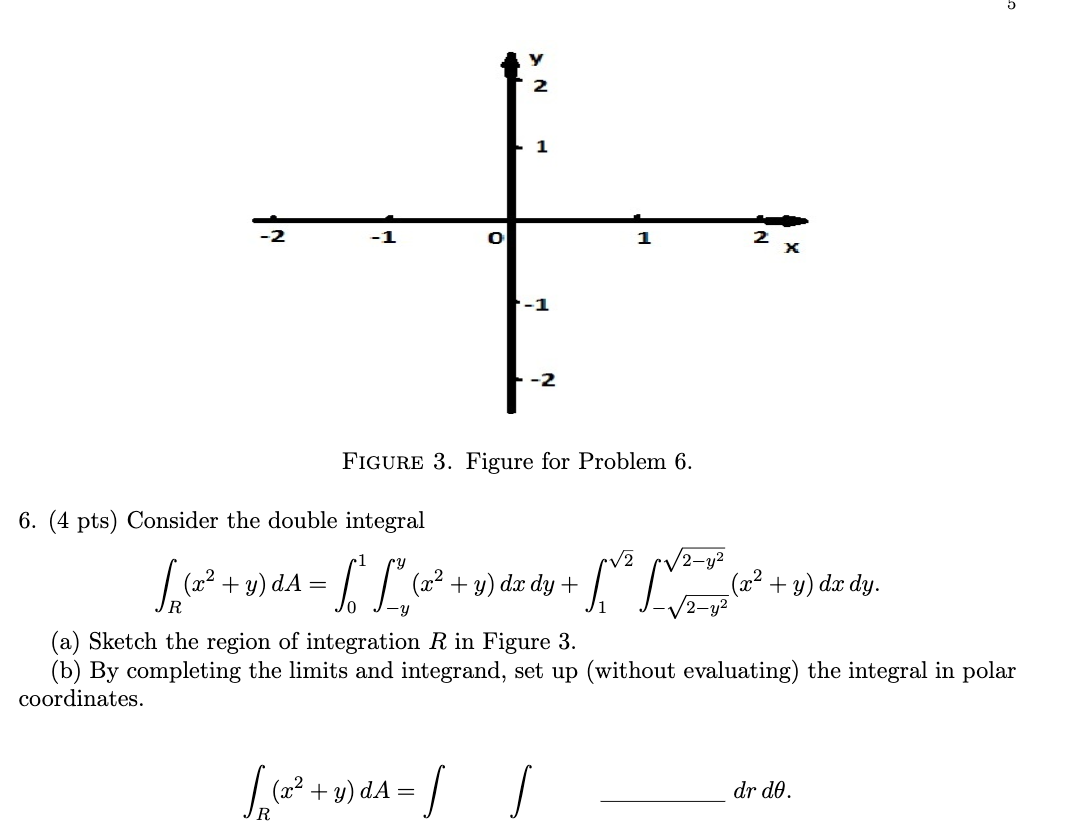1 -1 O 1 2 x FIGURE 3. Figure for Problem 6. 6. (4 pts) Consider the double integral 2 Spa (22 + y)da = [ L. (x2 + y) dx dy + √2-y² (x2 + y) dx dy. (a) Sketch the region of integration R in Figure 3. (b) By completing the limits and integrand, set up (without evaluating) the integral in polar coordinates. Sep (+2 +y)dA = dr do.

• ### 3. Draw the region D and evaluate the double integral using polar coordinates. dA, D= {(x,...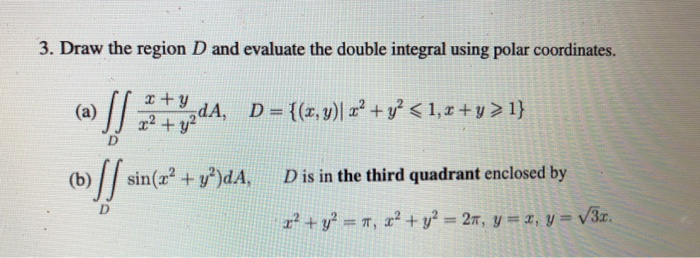3. Draw the region D and evaluate the double integral using polar coordinates. dA, D= {(x, y)| x2 + y² <1, x +y > 1} (b) sin(x2 + y2)dA, D is in the third quadrant enclosed by D r? + y2 = 7, x² + y2 = 24, y = 1, y = V3r.

• ### 7. (5 pts) By completing the limits and integrand, set up (without evaluating) an iterated inte-...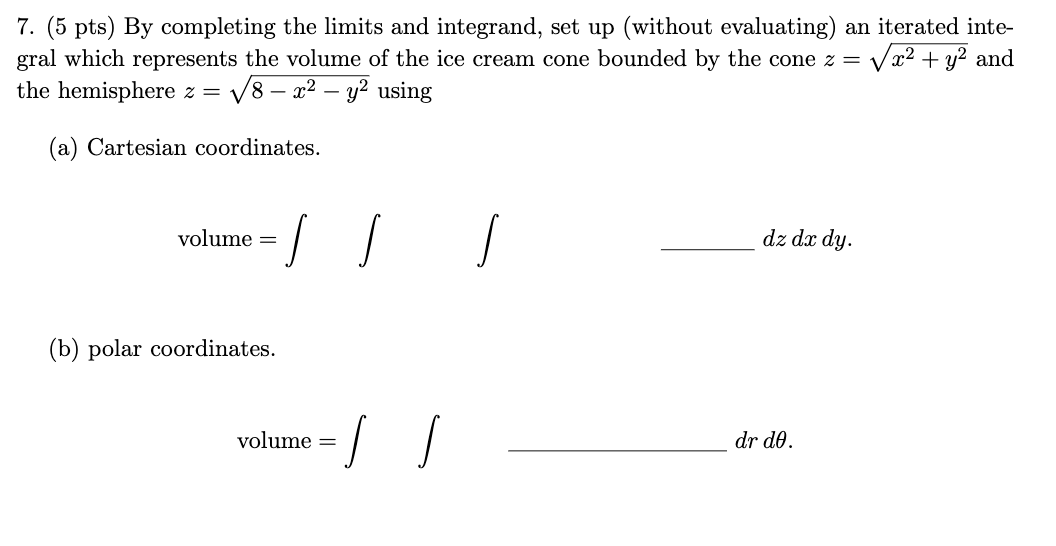7. (5 pts) By completing the limits and integrand, set up (without evaluating) an iterated inte- gral which represents the volume of the ice cream cone bounded by the cone z = V x2 + y2 and the hemisphere z = V8 – x2 - y2 using (a) Cartesian coordinates. volume = dz dx dy. (b) polar coordinates. volume = I dr de.

• ### 3. Consider the triple integral 2z sin(x2 + y2 +22 - 2x) dy da dz. Set...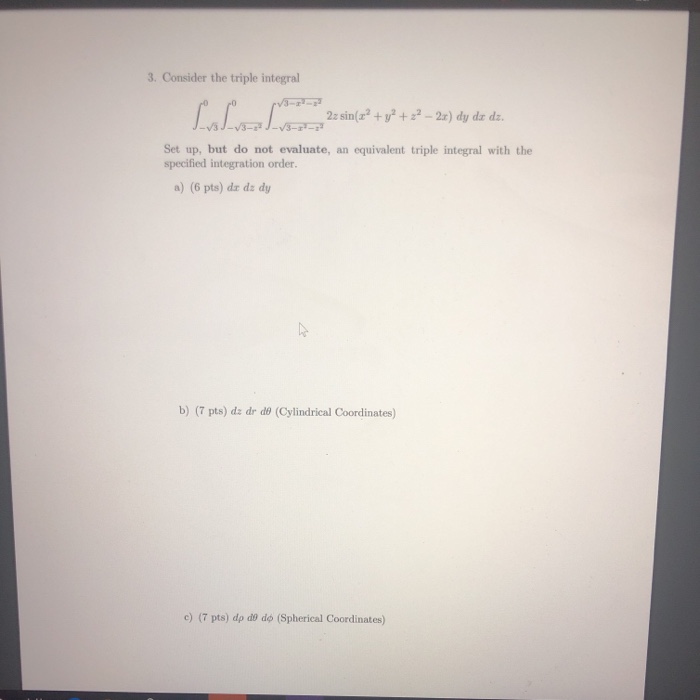3. Consider the triple integral 2z sin(x2 + y2 +22 - 2x) dy da dz. Set up, but do not evaluate, an equivalent triple integral with the specified integration order. a) (6 pts) da dz dy b) (7 pts) dz dr de (Cylindrical Coordinates) c) (7 pts) dp do do (Spherical Coordinates)

• ### The region R is bounded by the x-axis and y = V16 – x2 a) Sketch...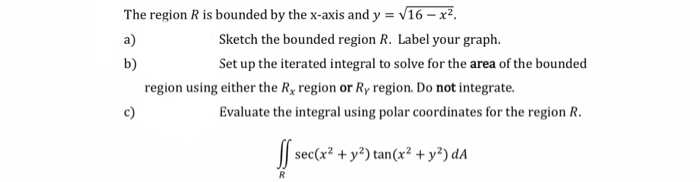The region R is bounded by the x-axis and y = V16 – x2 a) Sketch the bounded region R. Label your graph. b) Set up the iterated integral to solve for the area of the bounded region using either the Rx region or Ry region. Do not integrate. Evaluate the integral using polar coordinates for the region R. sec(x2 + y2) tan(x2 + y2) da c) R

• ### 7. (5 pts) By completing the limits and integrand, set up (without evaluating) an iterated inte-gral...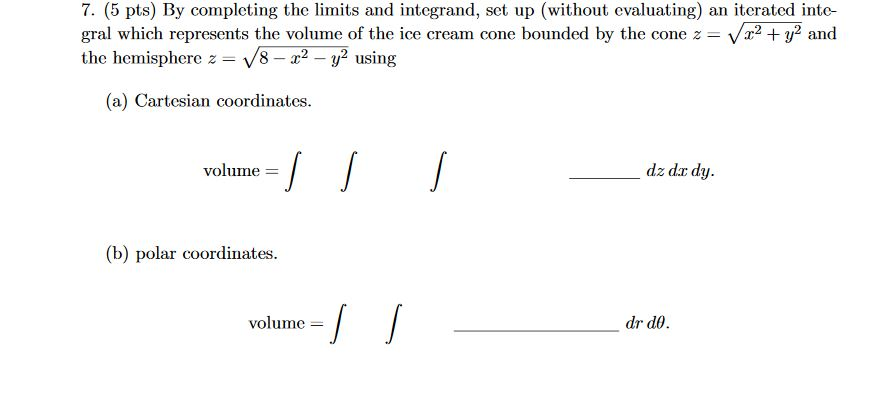7. (5 pts) By completing the limits and integrand, set up (without evaluating) an iterated inte-gral which represents the volume of the ice cream cone bounded by the cone z=√x2+y2andthe hemisphere z=√8−x2−y2using(a) Cartesian coordinates. 7. (5 pts) By completing the limits and integrand, set up (without evaluating) an iterated inte- gral which represents the volume of the ice cream cone bounded by the cone z = Vr2 + y2 and the hemisphere z = 18 - 22 - y2 using...

• ### (a) Evaluate the double integral 4. (sin cos y) dy dr. Hint: You may need the formula for integration by parts (b) Show...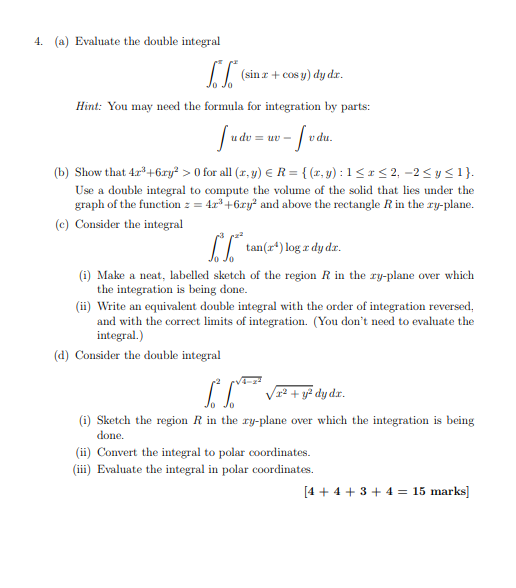(a) Evaluate the double integral 4. (sin cos y) dy dr. Hint: You may need the formula for integration by parts (b) Show that 4r+6ry>0 for all (r,y) ER-(x,y): 1S2,-2Sysi) Use a double integral to compute the volume of the solid that lies under the graph of the function 4+6ry and above the rectangle R in the ry-plane. e) Consider the integral tan(r) log a dyd. (i) Make a neat, labelled sketch of the region R in the ry-plane over...

• ### 2) The region R is bounded by the x-axis and y = V16 - x2 a)...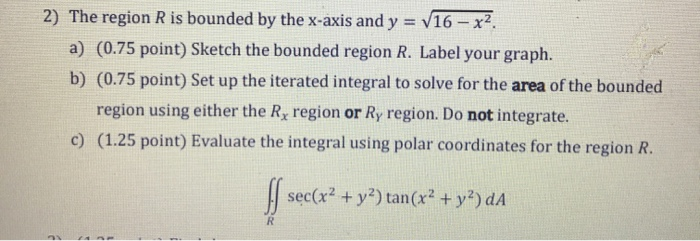2) The region R is bounded by the x-axis and y = V16 - x2 a) (0.75 point) Sketch the bounded region R. Label your graph. b) (0.75 point) Set up the iterated integral to solve for the area of the bounded region using either the Rx region or Ry region. Do not integrate. c) (1.25 point) Evaluate the integral using polar coordinates for the region R. sec(x2 + y2) tan(x2 + y2) dA R